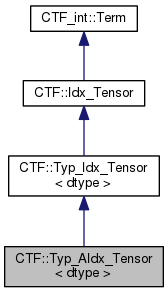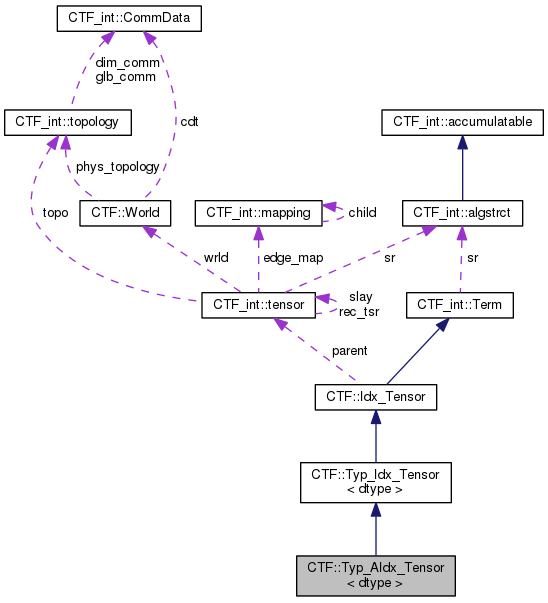Cyclops Tensor Framework parallel arithmetic on multidimensional arrays
CTF::Typ_AIdx_Tensor< dtype > Class Template Reference

`#include <idx_tensor.h>`

Inheritance diagram for CTF::Typ_AIdx_Tensor< dtype >:[legend]
Collaboration diagram for CTF::Typ_AIdx_Tensor< dtype >:[legend]

## Public Member Functions

Typ_AIdx_Tensor (Typ_Idx_Tensor< dtype > const &A)Public Member Functions inherited from CTF::Typ_Idx_Tensor< dtype >
~Typ_Idx_Tensor ()

Typ_Idx_Tensor (CTF_int::tensor *parent_, const char *idx_map_, int copy=0)
constructor takes in a parent tensor and its indices More...

Typ_Idx_Tensor (Typ_Idx_Tensor const &B, int copy=0, std::map< CTF_int::tensor *, CTF_int::tensor * > *remap=NULL)
copy constructor More...

Typ_Idx_Tensor< dtype > * tclone () const

CTF_int::Termclone (std::map< CTF_int::tensor *, CTF_int::tensor * > *remap=NULL) const
base classes must implement this copy function to retrieve pointer More...

void operator= (CTF_int::Term const &B)

void operator= (Idx_Tensor const &B)

void operator= (double scl)

void operator= (int64_t scl)

void operator= (int scl)

template<typename dtype_B >
Typ_Sum_Term< dtype, dtype_B > operator& (Typ_Idx_Tensor< dtype_B > B)

template<typename dtype_A , typename dtype_B >
Typ_Contract_Term< dtype_A, dtype_B, dtype > operator+= (Typ_Sum_Term< dtype_A, dtype_B > t)

template<typename dtype_A , typename dtype_B >
Typ_Contract_Term< dtype_A, dtype_B, dtype > operator= (Typ_Sum_Term< dtype_A, dtype_B > t)

Typ_AIdx_Tensor< dtype > operator~ ()

template<typename dtype_A >
Typ_Sum_Term< dtype_A, dtype > operator= (Typ_AIdx_Tensor< dtype_A > t)

template<typename dtype_A >
Typ_Sum_Term< dtype_A, dtype > operator+= (Typ_AIdx_Tensor< dtype_A > t)

void operator+= (CTF_int::Term const &B)

void operator+= (Idx_Tensor const &B)

void operator+= (double scl)

void operator+= (int64_t scl)

void operator+= (int scl)

void operator, (std::function< void(dtype &)> f)

void operator() (std::function< void(dtype &)> f)Public Member Functions inherited from CTF::Idx_Tensor
Idx_Tensor (CTF_int::tensor *parent_, const char *idx_map_, int copy=0)
constructor takes in a parent tensor and its indices More...

Idx_Tensor (CTF::Idx_Tensor const &other, int copy=0, std::map< CTF_int::tensor *, CTF_int::tensor * > *remap=NULL)
copy constructor More...

Idx_Tensor (CTF_int::algstrct const *sr)
constructor for scalar More...

Idx_Tensor (CTF_int::algstrct const *sr, double scl)

Idx_Tensor (CTF_int::algstrct const *sr, int64_t scl)

~Idx_Tensor ()

Idx_Tensor execute (std::vector< char > out_inds) const
evalues the expression to produce an intermediate with all expression indices remaining More...

void execute (Idx_Tensor output) const
evalues the expression, which just scales by default More...

double estimate_time (Idx_Tensor output) const
estimates the cost of a contraction More...

Idx_Tensor estimate_time (double &cost, std::vector< char > out_inds) const
estimates the cost the expression to produce an intermediate with all expression indices remaining More...

std::vector< char > get_uniq_inds () const
find list of unique indices that are involved in this term More...

void get_inputs (std::set< Idx_Tensor *, CTF_int::tensor_name_less > *inputs_set) const
appends the tensors this depends on to the input set More...

void operator= (CTF_int::Term const &B)
A = B, compute any operations on operand B and set. More...

void operator= (Idx_Tensor const &B)

void operator= (double scl)

void operator+= (double scl)

void operator-= (double scl)

void operator*= (double scl)

void multeq (double scl)

void operator= (int64_t scl)

void operator+= (int64_t scl)

void operator-= (int64_t scl)

void operator*= (int64_t scl)

void operator= (int scl)

void operator+= (int scl)

void operator-= (int scl)

void operator*= (int scl)

void operator+= (CTF_int::Term const &B)
A += B, compute any operations on operand B and add. More...

void operator-= (CTF_int::Term const &B)
A += B, compute any operations on operand B and add. More...

void operator*= (CTF_int::Term const &B)
negates term More...

Worldwhere_am_i () const
figures out what world this term lives on More...Public Member Functions inherited from CTF_int::Term
Term (algstrct const *sr)

virtual ~Term ()

virtual void get_inputs (std::set< CTF::Idx_Tensor *, tensor_name_less > *inputs_set) const =0
appends the tensors this depends on to the input set More...

void mult_scl (char const *mulscl)
multiply scaling factor by mulscl More...

Contract_Term operator* (Term const &A) const
constructs a new term which multiplies by tensor A More...

Contract_Term operator* (int64_t scl) const
multiples by a constant More...

Contract_Term operator* (double scl) const

Sum_Term operator+ (Term const &A) const
constructs a new term by addition of two terms More...

Sum_Term operator+ (double scl) const

Sum_Term operator+ (int64_t scl) const

Sum_Term operator- (Term const &A) const
constructs a new term by subtracting term A More...

Sum_Term operator- (double scl) const

Sum_Term operator- (int64_t scl) const

Termoperator- ()

void operator= (CTF::Idx_Tensor const &B)
A = B, compute any operations on operand B and set. More...

void operator= (Term const &B)

void operator+= (Term const &B)

void operator-= (Term const &B)

void operator*= (Term const &B)

void operator= (double scl)

void operator+= (double scl)

void operator<< (CTF_int::Term const &B)

void operator<< (double scl)

void operator-= (double scl)

void operator*= (double scl)

void operator= (int64_t scl)

void operator+= (int64_t scl)

void operator-= (int64_t scl)

void operator*= (int64_t scl)

void operator= (int scl)

void operator+= (int scl)

void operator-= (int scl)

void operator*= (int scl)

operator float () const
cast to float (works only if tensor type is castable to float) allows a scalar output More...

operator double () const
cast to double (works only if tensor type is castable to double) allows a scalar output More...

operator int64_t () const
cast to int64_t (works only if tensor type is castable to int64_t) allows a scalar output More...

operator int () const
cast to int64_t (works only if tensor type is castable to int64_t) allows a scalar output More...

## Additional Inherited MembersData Fields inherited from CTF::Idx_Tensor
CTF_int::tensorparent

char * idx_map

int is_intmData Fields inherited from CTF_int::Term
char * scale

algstrctsr

## Detailed Description

### template<typename dtype> class CTF::Typ_AIdx_Tensor< dtype >

Definition at line 224 of file idx_tensor.h.

## Constructor & Destructor Documentation

template<typename dtype>
 CTF::Typ_AIdx_Tensor< dtype >::Typ_AIdx_Tensor ( Typ_Idx_Tensor< dtype > const & A )
inline

Definition at line 322 of file idx_tensor.h.

The documentation for this class was generated from the following file: